A Statistical Model for Designers of Rate Monotonic Systems

Paul S. Heidmann

Computing Devices International

8800 Queen Av. S.

Bloomington, Mn 55431-1996

(612) 921-7377

paul.s.heidmann@cdev.com

# Introduction

In designing Rate Monotonic systems, developers have had difficulty predicting the schedulability of a given system during the design phase.  Typically, upper bounds (usually pessimistic) for the parameters of the Rate Monotonic equations are estimated based upon expected behavior.  If calculations then show that the (estimated) system will not be schedulable, designers modify the estimates in an attempt to ascertain what will be required to achieve schedulability.  This ad hoc approach has the disadvantages of assuming that the parameters are fixed and of providing the designer with little quantitative information.

The model presented in this paper provides a partial solution to these problems.  The model replaces the fixed parameter of task execution time with a statistical distribution in the Rate Monotonic equations.  Used in tandem with traditional Rate Monotonic calculations during the design phase provides quantitative information about the impact of varying execution times.  This information in turn may be used to perform risk analysis and schedulability impact studies.

Also presented in this paper is an application of this model to an example task set.  The effects of the perturbation of parameters are demonstrated.  Varying the means in the defining distributions is shown to have the greatest impact on schedulability.

# The Model in Detail

This section presents the model used in detail.  Motivation will be given for the modeling choices made.  The model will then be derived, with proofs given for the significant statements.

## Motivation for the Modeling Choices Made

In modeling the execution times of computer processes, care must be taken to allow for diverse behavior.  The variance of the execution time of each job with its inputs must be taken into account.  Furthermore, the characteristics of that variance will vary from process to process (e.g. the execution times of different processes may need to be modeled with different distributions).  It is also desirable to maintain some commonalty between the different distributions used to model the different processes as this simplifies computations and analysis.

To these ends, pieces of normal distributions have been chosen to model the execution times of tasks.  For each process, upper and lower bounds on the possible execution times are first chosen.  Next, a normal distribution (with distinct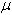and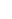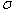) is chosen for the process.  The process's distribution is then defined to be that part of the normal distribution that lies between the upper and lower bounds (multiplied by a constant).

## Derivation of the Model

A task set is schedulable, by the Rate Monotonic Scheduling Theory, if the following condition is met:where the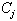's are the task execution times, the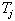's are the task periods, and the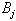's are the task blocking times.

Initially, the PDF (probability density function) for a piece of a normal distribution must be determined.  This amounts to deriving the appropriate constant,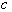, such that: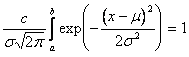.

Where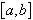is the desired piece of the normal distribution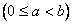with meanand variance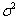.is then readily found:At this point, we have derived the PDF for this distribution: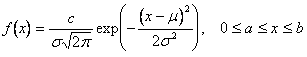The expected value of the random variable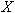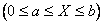described by this distribution can then be shown to be: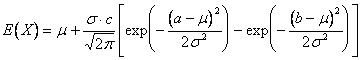.

Now, let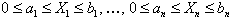be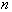stochastically independent random variables each described by the above distributions (e.g. each random variable is described by the above distribution with distinct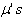,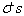,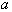's, and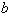's).  To determine the probability of schedulability, the PDF of the sum of these random variables is required.  Define the random variable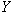as follows: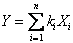,

where the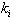's are positive.  It is now necessary to find the PDF for the random variable.  To this end, define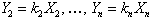.  These functions define a one to one transformation from the space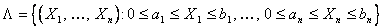to the space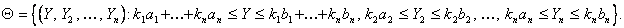Now define the following the following set of functions:These functions define a one to one transformation from the space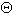to the space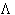.  Because of the assumption that task execution times are stochastically independent, the joint PDF of all task execution times will simply be: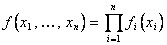,

where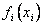is the PDF of the random variable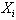.  Using a theorem from analysis (see [1, p. 288-289] for the two and three dimensional cases), we have the following: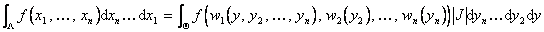,

where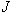is the Jacobian of the transformation defined by the functions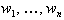.  In this case,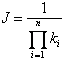.

This, however, gives the joint PDF of the random variables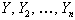:The extraneous variables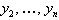can now be integrated out of the above joint PDF, giving the desired PDF of the variable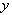.  To accomplish this, the limits of integration must first be determined.  To this end, define the following:Finally, the PDF of the desired variable,, can be stated:Using this PDF, the characteristics of a sum of random variables described by pieces of normal distributions is known.  It now remains to characterize the minimum of a collection of these sums.  Let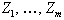be a collection of sums of random variables described by pieces of normal distributions.  Let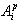be the event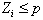, then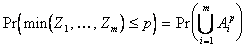.

The following lemma can now be used to find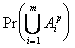:

Lemma:  Let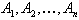beevents.  Then,Proof:  By induction.  Consider first the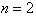case: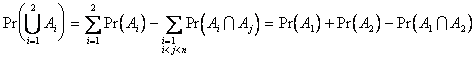.

Now, assume the above holds for; show the expression then holds for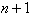:Apply the inductive hypothesis to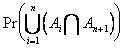: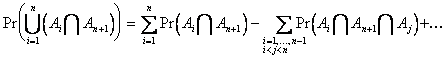Also apply the inductive hypothesis to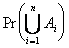.  Combining then gives the desired result.

By setting thes to the appropriate values, and computing the integrals numerically, the results presented here are used to predict schedulability and study the effects of parameter perturbations in a Rate Monotonic system.

# Parameter Perturbations

This section demonstrates the impact of the perturbation of the parameters that characterize task execution times on schedulability.  Specifically, a task set is defined with fixed bounds on the minimum and maximum execution time for each task.  The parameters defining how the task execution times are distributed between the respective minimum and maximum are then varied, and the effects shown.

The following task set is used in the example presented here:

 Task Min Exec Time Max Exec Time Period 1 38 42 100 2 38 42 150 3 98 102 350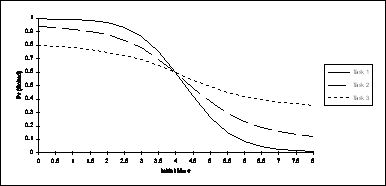First, the effect of varying the means of the normal distributions, pieces of which describe the execution times, is shown.  The graph should be interpreted as follows:  The line labeled Task 1 shows the probability of schedulability as the mean of the distribution describing task 1 is increased (along the x-axis) from an initial value ofto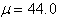.  While the mean of the distribution of task 1 is varied, the means of tasks 2 and 3 are held constant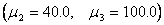.  The line labeled Task 2 shows the probability of schedulability as the mean of the distribution describing task 2 is varied fromto(while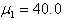and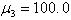).  Similarly, the line labeled Task 3 shows the probability of schedulability as the mean of the distribution describing task 3 varies from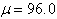to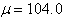(as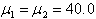are held constant).  The standard deviations of the distributions were held constant at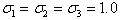.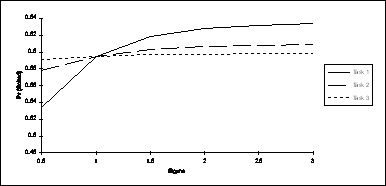Second, the effect of varying the standard deviations of the normal distributions, pieces of which describe the execution times, is shown.  This graph should be interpreted as follows:  The line labeled Task 1 is the probability of schedulability as the standard deviation of the distribution describing task 1 is varied (along the x-axis) from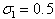to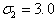.  While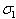is varied,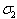and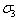are held constant at a value of 1.0.  Similarly, the lines labeled Task 2 and Task 3 show the effects of varyingand, both from 0.5 to 3.0, respectively (with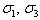and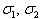held constant at 1.0, respectively).  The means of the distributions are held constant atand.

Using the techniques presented in this section, the developer is able to perform sensitivity analysis on a given task set.  This information may be used to provide risk analysis during the design phase, before the exact execution times are known.

# Future Research

This section discusses possible areas for future research.

Further development of the model is certainly required if it is to be as realistic as possible.  Blocking time could be modeled with the same types of distributions as execution times.  Interrupt arrivals could be modeled using a discrete distribution.  Sporadic server depletion could then be characterized.

Tasks with the capability of performing imprecise calculations (see ) may also be modeled.  Here, the model may be used to characterize average accuracy of the results of any given task.

# Conclusion

In this paper a paradigm for modeling Rate Monotonic systems is derived.  This paradigm is then used to demonstrate the effects of varying task execution time characteristics.  The means of the distributions are seen to have the greatest impact.  Standard deviations demonstrate some impact on schedulability, but it is seen to be rather minor in most cases.

Future development of the model will be required for complete characterization of most real-time systems.  The inclusion of blocking time will be required, as well as a method for modeling the arrival of interrupts.

# Bibliography

        Kaplan, Wilfred

        Liu, Jane W. S., Lin, Kwei-Jay, Natarajan, Swaminathan

Scheduling Real-Time, Periodic Jobs Using Imprecise Results

Proceedings of the Real-Time Systems Symposium, 1987

# Acknowledgment

The author wishes to thank Dwight Galster for his many helpful comments and suggestions.need not be between the upper and lower bounds of the process execution time being modeled.

This assumption is required for the methods of analysis that will be employed.  Notice that this assumption precludes the modeling of tasks that contend for common resources (other than processor time).

Notice that this value ofis below the minimum task execution time (38 units).  This models a task whose execution times tend to be closer to the minimum value.
Notice that this value ofis above the maximum task execution time (42 units).  This models a task whose execution times tend to be closer to the maximum value.